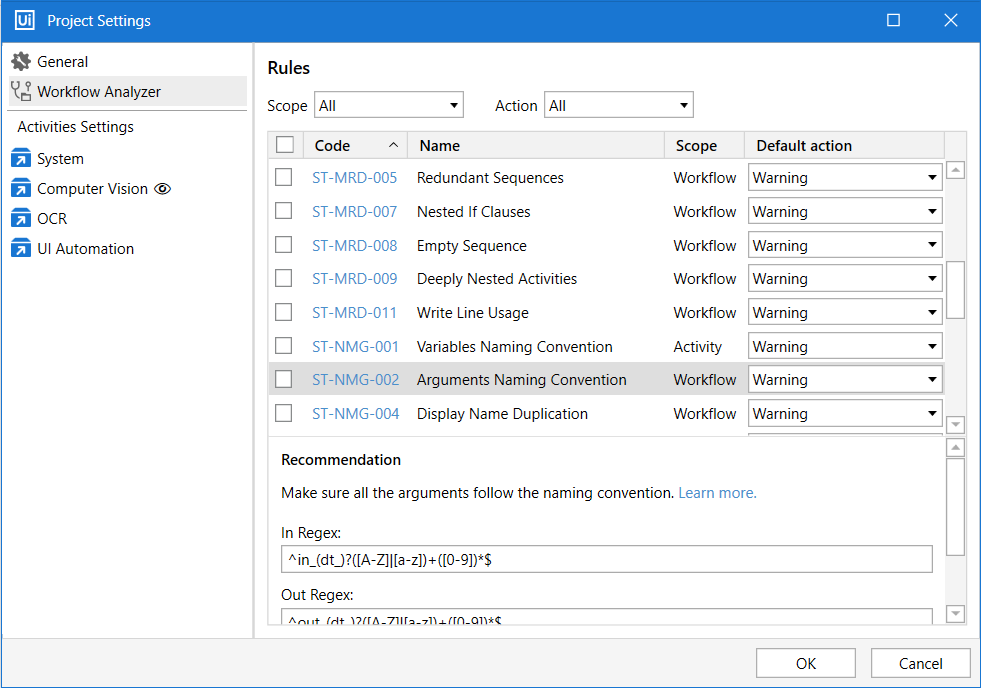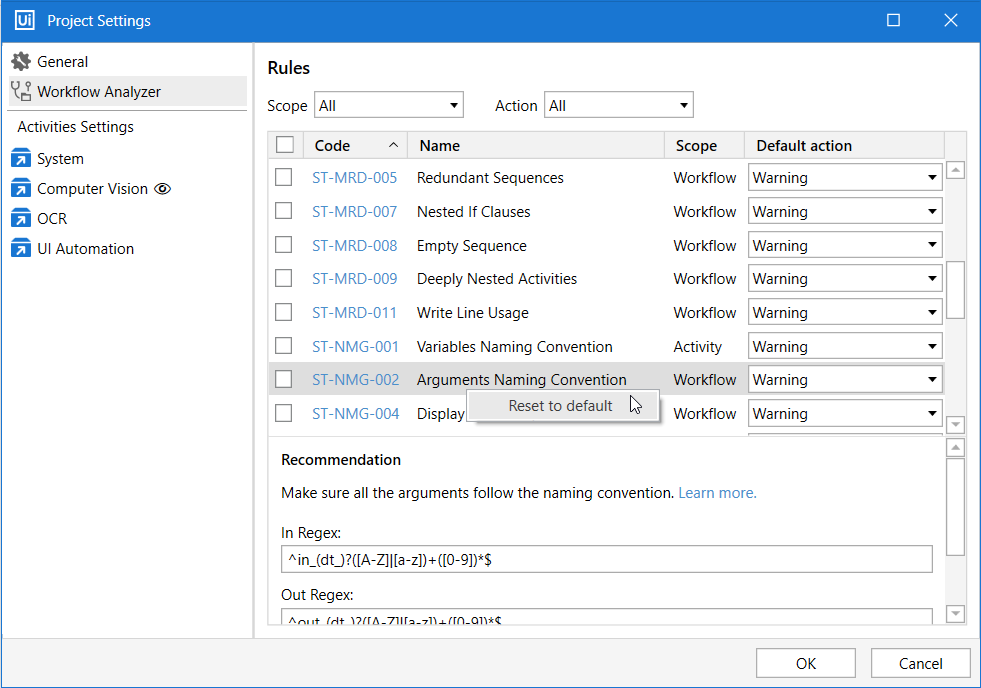Subscribe

# ST-NMG-002 - Arguments Naming Convention

Rule ID: `ST-NMG-002`

Scope: Workflow

# Description

This rule analyzes all arguments in the project and determines whether they follow the specific convention.

Arguments in the project should follow a specific naming convention to make it easier to understand the project and maintain it. The argument name should be meaningful and include an indication of its type:

• in – the argument can only be used within the given project.
• out – the argument can be used to pass data outside of a given project.
• io – the argument can be used both within and outside of a given project.

# Recommendation

Make sure all arguments follow the naming convention. The default Regex expression for this rule is:

• InRegex: `^in_(dt_)?([A-Z]|[a-z])+([0-9])*\$`
• OutRegex: `^out_(dt_)?([A-Z]|[a-z])+([0-9])*\$`
• InOutRegex: `^io_(dt_)?([A-Z]|[a-z])+([0-9])*\$`.

According to the above regex expression, the argument matches the expression if it starts with a prefix, followed by a lower or upper case letter, and a number.

For example, if the argument name in the workflow is `in_HelloWorld` then it is validated by the rule. Other examples of argument names that follow this regex rule are: `out_HelloWorld` and `io_Helloworld`.

# Modifying the Rule

In the Project Settings window, select the Workflow Analyzer tab. Find and select the rule, as in the image below:In the Regex section, add or remove characters from the search pattern. For example, if we remove the `[A-Z]` part of the expression for In arguments, the search pattern becomes `^in_(dt_)?([a-z])+([0-9])*\$`. Now the rule checks if In arguments start with a lower case letter after the prefix.

If we add `[a-z]|[A-Z])`, then the rule becomes `^in_(dt_)?([A-Z]|[a-z]|[a-z]|[A-Z])+([0-9])*\$`, and recognizes `in_HelloWonderfulWorld` as a valid In argument name.

# Examples of Regex Expressions

The default regex expression for this rule can be changed to another naming convention. Check the list below:

## Camel case

The camel case convention specifies that each word in the middle of the argument name begins with a capital letter, with no intervening spaces or punctuation.

Example of Regex expression: `^in_(dt_)?([A-Z]|[a-z]|[0-9])+([A-Z]|[a-z]|[0-9])`.
Valid argument names: `in_Hello1World2`, `in_helloWorld`, `in_Hello1World`.

## Pascal case

The Pascal case naming convention specifies that the argument name must contain concatenated capitalized words.

Example of Regex expression: `^in_(dt_)?([A-Z]|[0-9])+([A-Z]|[a-z]|[0-9])`.
Valid names: `in_Hello1World2`, `in_HelloWorld`, `in_Hello1World`.

## Kebab case

The Kebab case naming convention is similar to snake case, except that it replaces spaces with hyphens rather than underscores.

Example of Regex expression: `^in_(dt_)?([a-z]|[A-Z]|[0-9])+‐([a-z]|[A-Z]|[0-9])`
Valid names: `in_Hello1‐World2`, `in_Hello‐World`.

# Reset to Default Values

The default values for ST-NMG-002 Regex are:

• InRegex: `^in_(dt_)?([A-Z]|[a-z])+([0-9])*\$`
• OutRegex: `^out_(dt_)?([A-Z]|[a-z])+([0-9])*\$`
• InOutRegex: `^io_(dt_)?([A-Z]|[a-z])+([0-9])*\$`.

To reset these values to default right-click a rule in the Project Settings window, and then click Reset to default.Updated 2 years ago